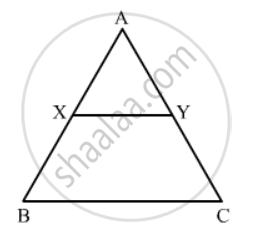Advertisement Remove all ads

# Xy is Drawn Parallel to the Base Bc of a ∆Abc Cutting Ab at X and Ac at Y. If Ab = 4 Bx and Yc = 2 Cm, Then Ay = (A) 2 Cm (B) 4 Cm (C) 6 Cm (D) 8 Cm - Mathematics

MCQ

XY is drawn parallel to the base BC of a ∆ABC cutting AB at X and AC at Y. If AB = 4 BX and YC = 2 cm, then AY =

#### Options

• 2 cm

• 4 cm

• 6 cm

• 8 cm

Advertisement Remove all ads

#### Solution

Given: XY is drawn parallel to the base BC of a ΔABC cutting AB at X and AC at Y. AB = 4BX and YC = 2 cm.

To find: AYIn ΔAXY and ΔABC,

$\angle AXY = \angle B \left( \text{Corresponding angles} \right)$
$\angle A = \angle A \left( \text{Common} \right)$
$\therefore ∆ AXY~ ∆ ABC \left( \text{AA similarity} \right)$

We know that if two triangles are similar, then their sides are proportional.
It is given that AB = 4BX.
Let AB = 4x and BX = x.

Then, AX = 3x

(AX)/(BX)=(AY)/(YC)

(3x)/(1x)=(AY)/2

AY=(3x xx2)/(1x)

AY= 6cm
Hence the correct answer is C

Concept: Triangles Examples and Solutions
Is there an error in this question or solution?
Advertisement Remove all ads

#### APPEARS IN

RD Sharma Class 10 Maths
Chapter 7 Triangles
Q 7 | Page 132
Advertisement Remove all ads

#### Video TutorialsVIEW ALL 

Advertisement Remove all ads
Share
Notifications

View all notifications

Forgot password?
Course